# 2.6.1.5.1 Quantitative uncertainty analysis

Page 22 of 41

The aim of the quantitative uncertainty analysis is to provide a probabilistic estimate of the change in the hydrological response variables due to coal resource development at the model nodes. A large number of parameter combinations are evaluated and, in line with the Approximate Bayesian Computation outlined in companion submethodology M09 (as listed in Table 1) for propagating uncertainty through models (Peeters et al., 2016), only those parameter combinations that result in acceptable model behaviour are accepted in the parameter ensemble used to make predictions.

Acceptable model behaviour is defined for each hydrological response variable based on the capability of the model to reproduce historical, observed time series of the hydrological response variable. For each hydrological response variable, a goodness of fit between model simulated and observed annual hydrological response variable is defined and an acceptance threshold defined.

The ensemble of predictions are the changes in hydrological response variable simulated with the parameter combinations for which the goodness of fit exceeds the acceptance threshold. The resulting ensembles are presented and discussed in Section 2.6.1.6.

## 2.6.1.5.1.1 Design of experiment

Table 5 lists the parameters used in the uncertainty analysis, the range and distribution type sampled in the design of experiment and the transformation of the parameter. These parameters and how they interact, is explained in detail the Australian Water Resources Assessment landscape model (AWRA-L) v4.5 documentation (Viney et al., 2015).

Table 5 AWRA-L parameters included in the quantitative uncertainty analysis

Parameter name

Units

Transformation

Minimum

Maximum

Prior distribution

cGsmax_hruDR

na

none

0.02

0.05

uniform

cGsmax_hruSR

na

none

0.001

0.05

uniform

ER_frac_ref_hruDR

na

none

0.04

0.25

uniform

FsoilEmax_hruDR

na

none

0.2

1

uniform

FsoilEmax_hruSR

na

none

0.2

1

uniform

K_gw_scale

na

log10

0.001

1

uniform

K0sat_scale

na

log10

0.1

10

uniform

Kdsat_scale

na

log10

0.01

1

uniform

Kssat_scale

na

log10

0.0001

0.1

uniform

Pref_gridscale

na

none

0.1

5

uniform

S0max_scale

na

none

0.5

5

uniform

Sdmax_scale

na

none

0.5

1

uniform

Ssmax_scale

na

none

0.5

3

uniform

Ud0_hruDR

mm/d

log10

0.001

10

uniform

AWRA-L = Australian Water Resources Assessment landscape model; na = data not applicable

Through a space-filling Latin Hypercube sampling (Santer et al., 2003), 10,000 parameter combinations are generated of the AWRA-L parameters, with the ranges and transformations shown in Table 5. These ranges and transforms are chosen by the modelling team based on previous experience in regional and continental calibration of AWRA-L (Viney et al., 2015). These mostly correspond to the upper and lower limits of each parameter during calibration.

Each of the 10,000 parameter sets is used to drive AWRA-L to generate streamflow time series at each 0.05 x 0.05 degrees grid cell (Jones et al., 2009). The results of these runs are combined with the change in surface watergroundwater flux simulated with the groundwater model (companion product 2.6.2, Cui et al., 2016), as outlined in Section 2.6.1.1, to create time series of total streamflow under baseflow and coal resource development pathway (CRDP) conditions. This resulted in a database of 3756 evaluated parameter combinations for which historical streamflow as well as streamflow in baseline and CRDP are available. While the coverage of parameter space is limited with less than 4000 simulations, visual inspection of the successfully evaluated parameters showed that there was adequate coverage of all parameters and no bias or gaps in the sampling of the parameter hyperspace.

## 2.6.1.5.1.2 Observations

For 10 of the 16 model nodes in the model domain, observations of streamflow are available. For these catchments the historical observations of streamflow are summarised into the nine hydrological response variables for all years with a full observational record. The equivalent historical simulated hydrological response variable values are computed from the design of experiment runs. The difference between these observed and simulated historical hydrological response variable values are used in the Approximate Bayesian Monte Carlo analysis to select behavioural parameter combinations.

The difference between simulated and observed historical hydrological response variables is summarised as the average of a modified index of agreement (Willmott, 1981):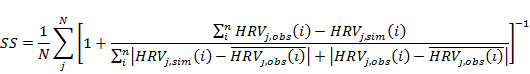(1)

with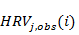the observed hydrological response variable in catchment(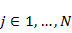) for year(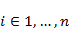).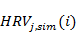is the equivalent simulated value. The summary statistic varies between 0.5 and 1, where 1 represents a perfect fit.

## 2.6.1.5.1.3 Predictions

A total of 3756 time series with a length of 90 years of hydrological response variable values are available for baseline,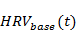, and CRDP conditions,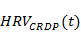.

These two time series are summarised through the maximum raw change, amax, the maximum percent change, pmax, and the year of maximum change, tmax. The percentage change is defined as: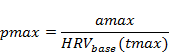(2)

In other words, it is the maximum change in hydrological response variable divided by the value of the hydrological response variable under baseline conditions in the year when the maximum change occurs. The pmax value is very useful to contextualise changes in flux estimates. It can lead to aberrant results, especially for hydrological response variables that are categorical (such as the number of low-flow days) or when the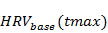is very small or zero.

## 2.6.1.5.1.4 Sensitivity analysis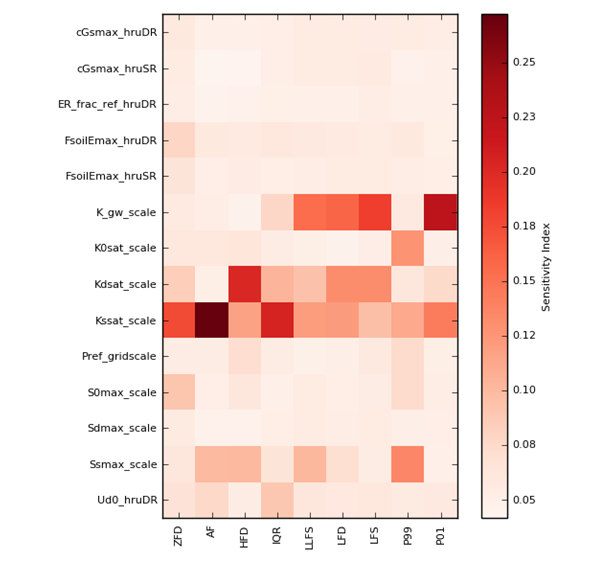Figure 9 Sensitivity indices of hydrological response variable summary statistics to AWRA-L parameters

Sensitivity indices are a relative measure, darker colors indicate more sensitive hydrological response variable/parameter pairs

Figure 9 shows the sensitivity indices of the summary statistics for hydrological response variable to each parameter from Table 5, computed with the density-based sensitivity analysis methodology of Plischke et al. (2013).

From this sensitivity analysis it is clear that the hydrological response variables are sensitive to different parameters. For the metrics that are affected by high-streamflow, such as annual flow (AF), interquartile range (IQR), number of flood (high-flow) days (FD) and daily streamflow at the 99th percentile (P99), the most important parameters are those controlling the quick-flow and interflow components of the hydrograph (K0sat_scale, Kssat_scale and to a lesser extent Ssmax_scale, see Viney et al., 2015). For the hydrological response variables that are most sensitive to low-streamflow, daily streamflow at the the 1st percentile (P01), the number of low-flow days (LFD), the number of low-flow spells (LFS), the length of the longest low-flow spell (LLFS), and the zero-flow days (ZFD), are most sensitive to K_gw_scale, which controls the slow-flow component of the simulated hydrograph (Viney et al., 2015).

The sensitivity analysis highlights the importance of constraining parameters with observations of the same type as the prediction. For instance, observations of annual flow will mainly constrain Kssat_scale, but will not be able to constrain K_gw_scale, which is the most important parameter for low-flow predictions.

## 2.6.1.5.1.5 Selection of behavioural parameter combinations

A central concept in the Approximate Bayesian Computation methodology, outlined in companion submethodology M09 (as listed in Table 1) for propagating uncertainty through models (Peeters et al., 2016), is the summary statistic and corresponding rejection threshold. The summary statistic is a single number that quantifies the performance of the model with a given parameter combination. The rejection threshold is the minimum value this summary statistic needs to be for a proposed parameter combination to be accepted in the posterior parameter distribution for the hydrological response variable.

In the selection of behavioural parameter combinations for each hydrological response variable, three criteria and thresholds are used:

1. hydrological response variable specific summary statistic based on the average Nash–Sutcliffe efficiency (Eq. 1)
2. simulated rate of extraction for coal seam gas production by the groundwater model
3. average historical surface watergroundwater flux at Casino in September and October.

The first criterion constrains the AWRA-L parameters, while the second and third constrain the change in surface water groundwater flux from the groundwater model. These two criteria and acceptance thresholds are discussed in greater detail in companion product 2.6.2 (Cui et al., 2016).

The acceptance threshold for the first criterion is set to the 75th percentile of the design of experiment simulations. In other words, the 25% best parameter combinations for that hydrological response variable are accepted.

Only the parameter combinations that meet the thresholds for all three criteria are accepted. The predicted change in the hydrological response variables corresponding to the behavioural parameter combinations are presented in Section 2.6.1.6. Before presenting and discussing these results, the main assumptions and model choices underpinning the predictions are discussed in the qualitative uncertainty analysis.

Last updated:
7 January 2019